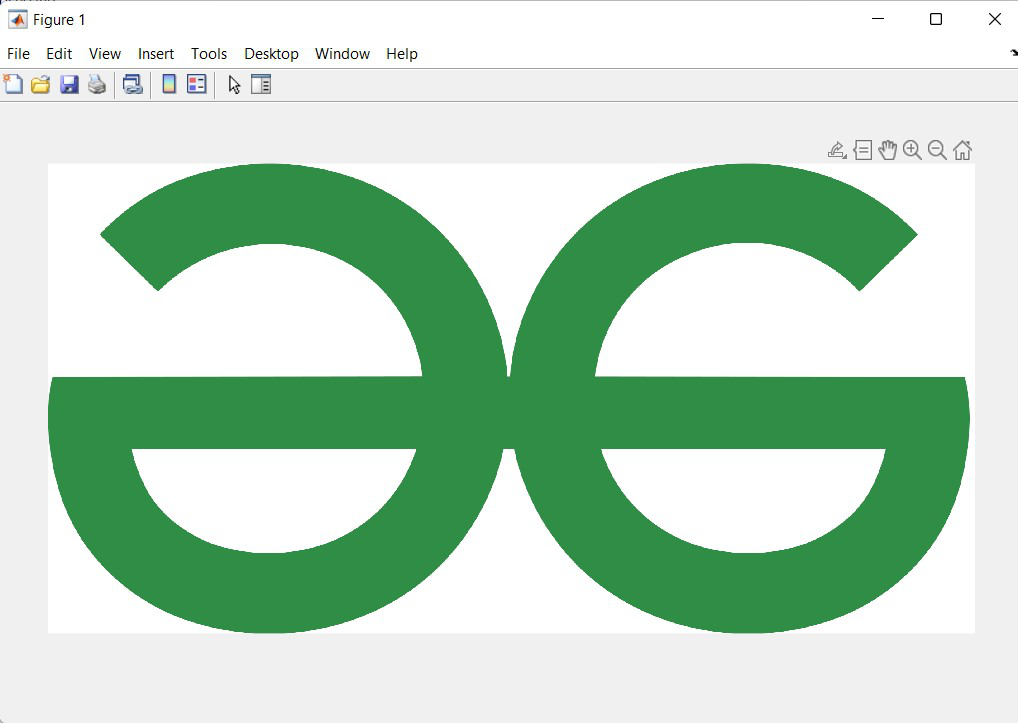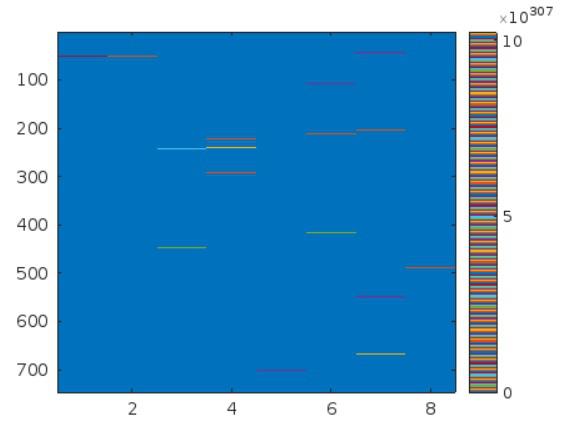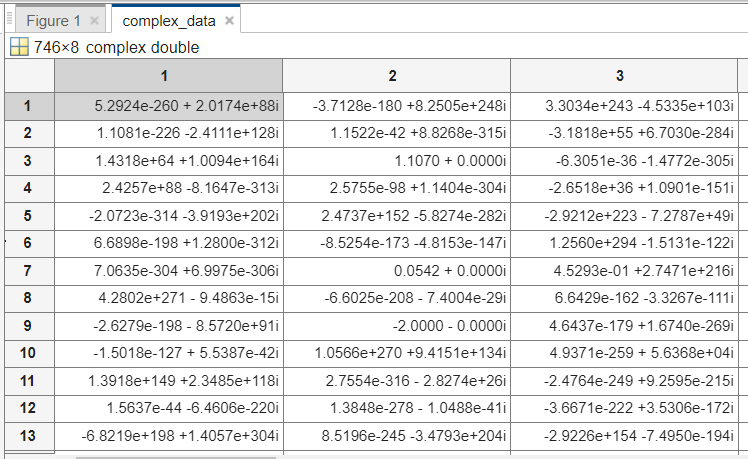GeeksforGeeks App
Open AppBrowser
Continue

# How to Read Image File or Complex Image File in MATLAB?

MATLAB is a programming and numeric computing platform used by millions of engineers and scientists to analyze data, develop algorithms, and create models. For Image Reading in MATLAB, we use the image processing toolbox. In this ToolBox, there are many methods such as imread(), imshow() etc.

• imshow(I) : shows the grayscale image I in a figure.
• imshow(I,[low high]) : shows the grayscale image I, specifying the display range as a two-element vector.
• imshow(RGB) : shows the truecolor image RGB in a figure.
• imshow(BW) : shows the binary image BW in a figure. For 0 pixel will be black and for 1 pixel will be white.
• imshow(filename) : shows the image stored in the graphics file specified by filename.
• himage = imshow(___) : returns the image object created by imshow.

Example 1:

## Matlab

 `% MATLAB Simple code for Reading and Displaying Image``img = imread(``'GeeksforGeeks.png'``); `` ` `% This will Create a matrix named img ``% And store image in form of 3D matrix ``% in the format of RGB`` ` `imshow(img)        `` ``% Displaying the image in new Window`

Output:### Read a Complex Images in MATLAB:

For reading a  complex image in MATLAB, the real and imaginary parts of the pixel will be stored adjacent to each other. All the coherent systems generate complex data such as Synthetic Aperture Radar images, Ultrasound images, etc.

Example 2:

## Matlab

 `% MATLAB code for read a complex image``% For open an image``GFG=fopen(``'GeeksforGeeks.png'``,``'r'``);`` ` `% To read an image``full_data=fread(GFG,[746*2 3680],``'double'``);`` ` `% To close an image``fclose(GFG);``R_data=full_data(1:2:``end``,:);``I_data=full_data(2:2:``end``,:);``complex_data=complex(R_data,I_data);``figure,imagesc(abs(complex_data));``colormap(rgbplot);`

Output:Complex Data Matrix After Execution:My Personal Notes arrow_drop_up